Home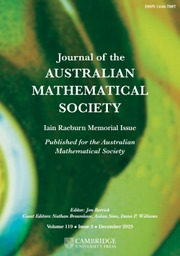Journal of the Australian Mathematical Society

# UNIQUENESS OF SOLUTIONS TO SCHRÖDINGER EQUATIONS ON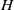$H$ -TYPE GROUPS

Published online by Cambridge University Press:  07 August 2013

Corresponding

## Abstract

This paper deals with the Schrödinger equation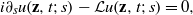$i{\partial }_{s} u(\mathbf{z} , t; s)- \mathcal{L} u(\mathbf{z} , t; s)= 0,$ where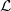$\mathcal{L}$ is the sub-Laplacian on the Heisenberg group. Assume that the initial data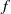$f$ satisfies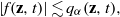$\vert f(\mathbf{z} , t)\vert \lesssim {q}_{\alpha } (\mathbf{z} , t),$ where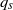${q}_{s}$ is the heat kernel associated to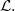$\mathcal{L} .$ If in addition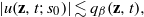$\vert u(\mathbf{z} , t; {s}_{0} )\vert \lesssim {q}_{\beta } (\mathbf{z} , t),$ for some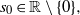${s}_{0} \in \mathbb{R} \setminus \{ 0\} ,$ then we prove that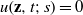$u(\mathbf{z} , t; s)= 0$ for all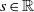$s\in \mathbb{R}$ whenever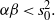$\alpha \beta \lt { s}_{0}^{2} .$ This result holds true in the more general context of$H$ -type groups. We also prove an analogous result for the Grushin operator on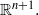${ \mathbb{R} }^{n+ 1} .$

## MSC classification

Type
Research Article
Information
Journal of the Australian Mathematical Society , December 2013 , pp. 297 - 314

## References

Berndt, J., Tricerri, F. and Vanhecke, L., Generalized Heisenberg Groups and Damek–Ricci Harmonic Spaces, Lecture Notes in Mathematics, 1598 (Springer, Berlin, 1995).CrossRefGoogle Scholar
Chanillo, S., ‘Uniqueness of solutions to Schrödinger equations on complex semi-simple Lie groups’, Proc. Indian Acad. Sci. Math. Sci. 117 (2007), 325331.CrossRefGoogle Scholar
Cowling, M., Escauriaza, L., Kenig, C. E., Ponce, G. and Vega, L., ‘The Hardy uncertainty principle revisted’, Indiana Univ. Math. J. 59 (6) (2010), 20072026.CrossRefGoogle Scholar
Escauriaza, L., Kenig, C. E., Ponce, G. and Vega, L., ‘Hardy’s uncertainty principle, convexity and Schrödinger evolutions’, J. Eur. Math. Soc. (JEMS) 10 (2008), 883907.CrossRefGoogle Scholar
Escauriaza, L., Kenig, C. E., Ponce, G. and Vega, L., ‘Convexity of free solutions of Schrödinger equations with Gaussian decay’, Math. Res. Lett. 15 (2008), 957971.CrossRefGoogle Scholar
Escauriaza, L., Kenig, C. E., Ponce, G. and Vega, L., ‘The sharp Hardy uncertainty principle for Schrödinger evolutions’, Duke Math. J. 155 (2010), 163187.CrossRefGoogle Scholar
Escauriaza, L., Kenig, C. E., Ponce, G. and Vega, L., ‘Uncertainty principles of Morgan type and Schrödinger evolutions’, J. Lond. Math. Soc. (2) 83 (2011), 187207.CrossRefGoogle Scholar
Ionescu, I. D. and Kenig, C. E., ‘Uniqueness properties of solutions of Schrödinger equations’, J. Funct. Anal. 232 (2006), 90136.CrossRefGoogle Scholar
Kaplan, A., ‘Fundamental solutions for a class of hypoelliptic PDE generated by composition of quadratic forms’, Trans. Amer. Math. Soc. 258 (1980), 147153.CrossRefGoogle Scholar
Kenig, C. E., Ponce, G. and Vega, L., ‘On the support of solutions of nonlinear Schrödinger equations’, Comm. Pure Appl. Math. 56 (2002), 12471262.CrossRefGoogle Scholar
Ludwig, J. and Müller, D., ‘Uniqueness of solutions to Schrödinger equations on 2-step nilpotent Lie groups’, 2012, arXiv:1207.4652.Google Scholar
Pasquale, A. and Sundari, M., ‘Uncertainty principles for the Schrödinger equation on Riemannian symmetric spaces of the noncompact type’, Ann. Inst. Fourier (Grenoble) 62 (2012), 859886.CrossRefGoogle Scholar
Randall, J., ‘The heat kernel for generalized Heisenberg groups’, J. Geom. Anal. 6 (1996), 287316.CrossRefGoogle Scholar
Ricci, F., ‘Commutative algebras of invariant functions on groups of Heisenberg type’, J. Lond. Math. Soc. 32 (1985), 265271.CrossRefGoogle Scholar
Robbiano, L., ‘Unicité forte à l’infini pour KdV’, ESAIM Control Optim. Calc. Var. 8 (2002), 933939.CrossRefGoogle Scholar
Thangavelu, S., Lectures on Hermite and Laguerre expansions, Mathematical Notes, 42 (Princeton University Press, Princeton, 1993).Google Scholar
Thangavelu, S., An Introduction to the Uncertainty Principle. Hardy’s Theorem on Lie Groups, Progress in Mathematics, 217 (Birkhäuser, Boston, MA, 2004).CrossRefGoogle Scholar
Tuan, V.-K., ‘Uncertainty principles for the Hankel transform’, Integral Transforms Spec. Funct. 18 (2007), 369381.CrossRefGoogle Scholar
Zhang, B.-Y., ‘Unique continuation for the Korteweg-de Vries equation’, SIAM J. Math. Anal. 23 (1992), 5571.CrossRefGoogle Scholar

### Full text views

Full text views reflects PDF downloads, PDFs sent to Google Drive, Dropbox and Kindle and HTML full text views.

Total number of HTML views: 0
Total number of PDF views: 130 *
View data table for this chart

* Views captured on Cambridge Core between September 2016 - 23rd January 2021. This data will be updated every 24 hours.

Access
Hostname: page-component-76cb886bbf-7fh6l Total loading time: 0.529 Render date: 2021-01-23T21:49:42.100Z Query parameters: { "hasAccess": "1", "openAccess": "0", "isLogged": "0", "lang": "en" } Feature Flags: { "shouldUseShareProductTool": true, "shouldUseHypothesis": true, "isUnsiloEnabled": true, "metricsAbstractViews": false, "figures": false, "newCiteModal": false }

# Send article to Kindle

Note you can select to send to either the @free.kindle.com or @kindle.com variations. ‘@free.kindle.com’ emails are free but can only be sent to your device when it is connected to wi-fi. ‘@kindle.com’ emails can be delivered even when you are not connected to wi-fi, but note that service fees apply.

Find out more about the Kindle Personal Document Service.

UNIQUENESS OF SOLUTIONS TO SCHRÖDINGER EQUATIONS ON$H$-TYPE GROUPS Available formats × # Send article to Dropbox To send this article to your Dropbox account, please select one or more formats and confirm that you agree to abide by our usage policies. If this is the first time you use this feature, you will be asked to authorise Cambridge Core to connect with your <service> account. Find out more about sending content to Dropbox. UNIQUENESS OF SOLUTIONS TO SCHRÖDINGER EQUATIONS ON$H$ -TYPE GROUPS
Available formats
×

# Send article to Google Drive

To send this article to your Google Drive account, please select one or more formats and confirm that you agree to abide by our usage policies. If this is the first time you use this feature, you will be asked to authorise Cambridge Core to connect with your <service> account. Find out more about sending content to Google Drive.

UNIQUENESS OF SOLUTIONS TO SCHRÖDINGER EQUATIONS ON$H\$ -TYPE GROUPS
Available formats
×
×